Test: The Bipolar Junction Transistor

# Test: The Bipolar Junction Transistor

Test Description

## 20 Questions MCQ Test GATE Electrical Engineering (EE) 2023 Mock Test Series | Test: The Bipolar Junction Transistor

Test: The Bipolar Junction Transistor for Electronics and Communication Engineering (ECE) 2022 is part of GATE Electrical Engineering (EE) 2023 Mock Test Series preparation. The Test: The Bipolar Junction Transistor questions and answers have been prepared according to the Electronics and Communication Engineering (ECE) exam syllabus.The Test: The Bipolar Junction Transistor MCQs are made for Electronics and Communication Engineering (ECE) 2022 Exam. Find important definitions, questions, notes, meanings, examples, exercises, MCQs and online tests for Test: The Bipolar Junction Transistor below.
Solutions of Test: The Bipolar Junction Transistor questions in English are available as part of our GATE Electrical Engineering (EE) 2023 Mock Test Series for Electronics and Communication Engineering (ECE) & Test: The Bipolar Junction Transistor solutions in Hindi for GATE Electrical Engineering (EE) 2023 Mock Test Series course. Download more important topics, notes, lectures and mock test series for Electronics and Communication Engineering (ECE) Exam by signing up for free. Attempt Test: The Bipolar Junction Transistor | 20 questions in 60 minutes | Mock test for Electronics and Communication Engineering (ECE) preparation | Free important questions MCQ to study GATE Electrical Engineering (EE) 2023 Mock Test Series for Electronics and Communication Engineering (ECE) Exam | Download free PDF with solutions
 1 Crore+ students have signed up on EduRev. Have you?
Test: The Bipolar Junction Transistor - Question 1

### A uniformly doped silicon pnp transistor is biased in the forward-active mode. The doping profile is NE = 108 cm-3, NB = 5.2 x 1016 cm-3 and NC = 1015  cm-3 .For VEB = 0.6 V, the pB at x =0 is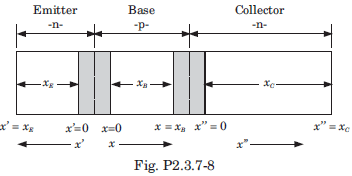Detailed Solution for Test: The Bipolar Junction Transistor - Question 1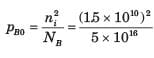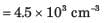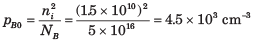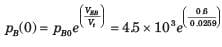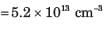Test: The Bipolar Junction Transistor - Question 2

### An npn bipolar transistor having uniform doping of NE = 1018 cm-3  NB = 1016 cm-3 and Nc = 6 x 1015 cm-3 is operating in the inverse-active mode with VBE = - 2 V and VBC = 0.6 V. The geometry of transistor is shown in fig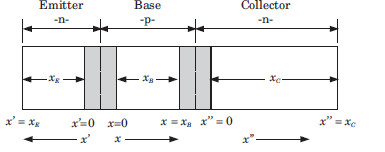Q. The minority carrier concentration at x =  xB is

Detailed Solution for Test: The Bipolar Junction Transistor - Question 2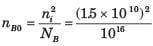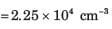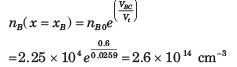Test: The Bipolar Junction Transistor - Question 3

### An npn bipolar transistor having uniform doping of NE = 1018 cm-3  NB = 1016 cm-3 and Nc = 6 x 1015 cm-3 is operating in the inverse-active mode with VBE = - 2 V and VBC = 0.6 V. The geometry of transistor is shown in figQ. The minority carrier concentration at x" = 0 is

Detailed Solution for Test: The Bipolar Junction Transistor - Question 3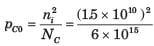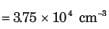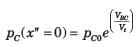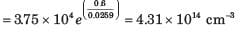Test: The Bipolar Junction Transistor - Question 4

An pnp bipolar transistor has uniform doping of NE = 6 x 1017 cm-3, N= 2 x 1016  cm-3 and N= 5 x 1014 cm-3. The transistor is operating is inverse-active mode. The maximum VCB voltage, so that the low injection condition applies, is

Detailed Solution for Test: The Bipolar Junction Transistor - Question 4

Low injection limit is reached when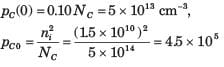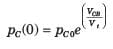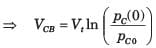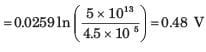Test: The Bipolar Junction Transistor - Question 5

The following currents are measured in a uniformly doped npn bipolar transistor:

InE  = 1.20 mA, IpE  = 0.10 mA, InC = 1.18 mA
IR = 0.20 mA, IG = 1 μA, IpC0 = 1 μA

Q.

The α is

Detailed Solution for Test: The Bipolar Junction Transistor - Question 5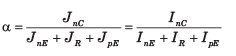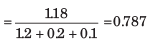Test: The Bipolar Junction Transistor - Question 6

The following currents are measured in a uniformly doped npn bipolar transistor:

InE  = 1.20 mA, IpE  = 0.10 mA, InC = 1.18 mA
IR = 0.20 mA, IG = 1 μA, IpC0 = 1 μA

Q.

The β is

Detailed Solution for Test: The Bipolar Junction Transistor - Question 6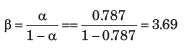Test: The Bipolar Junction Transistor - Question 7

The following currents are measured in a uniformly doped npn bipolar transistor:

InE  = 1.20 mA, IpE  = 0.10 mA, InC = 1.18 mA
IR = 0.20 mA, IG = 1 μA, IpC0 = 1 μA

Q.

The  γ is

Detailed Solution for Test: The Bipolar Junction Transistor - Question 7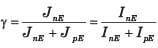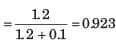Test: The Bipolar Junction Transistor - Question 8

A silicon npn bipolar transistor has doping concentration of NE = 2 x 1018cm-3, NB =1017cm-3 and N = 15 x 1016 cm-3. The area is 10-3  cm2 and neutral base width is 1 μm. The transistor is biased in the active region at VBE = 0.5 V. The collector current is
(DB = 20 cm2/s)

Detailed Solution for Test: The Bipolar Junction Transistor - Question 8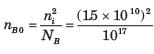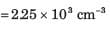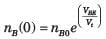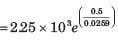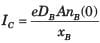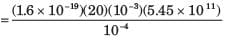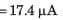Test: The Bipolar Junction Transistor - Question 9

A uniformly doped npn bipolar transistor has following parameters:

NE = 1018 cm-3 NB = 5 x 1016 cm-3,
Nc = 2 x 1019 cm-3,
DE = 8 cm2 /s , DB = 15 cm2 /s , Dc = 14 cm2 /s
xE = 0.8 μm, xB = 0.7 μm

The emitter injection efficiency γ is

Detailed Solution for Test: The Bipolar Junction Transistor - Question 9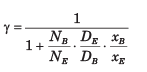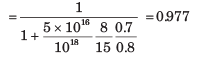Test: The Bipolar Junction Transistor - Question 10

In bipolar transistor biased in the forward-active region the base current is IB = 50 μA. and the collector currents is IC = 27 μA. The α is

Detailed Solution for Test: The Bipolar Junction Transistor - Question 10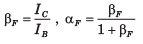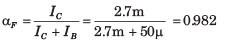Test: The Bipolar Junction Transistor - Question 11

For the transistor in fig., IS = 1015 A, βF = 100,  βR = 1. The current ICBO is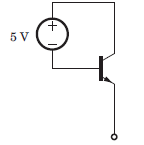Detailed Solution for Test: The Bipolar Junction Transistor - Question 11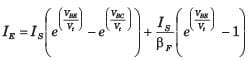= 0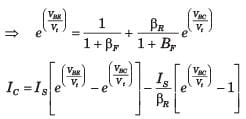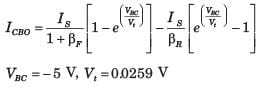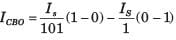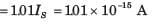Test: The Bipolar Junction Transistor - Question 12

Determine the region of operation for the transistor shown in circuit in question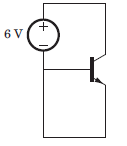Detailed Solution for Test: The Bipolar Junction Transistor - Question 12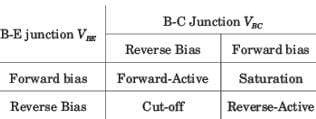VBE = 0 , VBC < 0, Thus both junction are in reverse bias. Hence cut off region.

Test: The Bipolar Junction Transistor - Question 13

Determine the region of operation for the transistor shown in circuit in question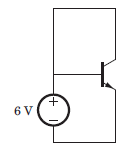Detailed Solution for Test: The Bipolar Junction Transistor - Question 13

VBE > 0, VBC = 0, Base-Emitter junction forward bais, Base-collector junction reverse bias, Hence forward-active region.

Test: The Bipolar Junction Transistor - Question 14

Determine the region of operation for the transistor shown in circuit in question.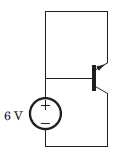Detailed Solution for Test: The Bipolar Junction Transistor - Question 14

VBE = 0, VBC> 0, Base-Emitter junction reverse bais , Base-collector junction forward bias, Hence reverse-active region.

Test: The Bipolar Junction Transistor - Question 15

Determine the region of operation for the transistor shown in circuit in question.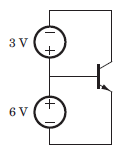Detailed Solution for Test: The Bipolar Junction Transistor - Question 15

VBE = 6V, VBC  = 3V Both junction are forward biase, Hence saturation region.

Test: The Bipolar Junction Transistor - Question 16

For the circuit shown in fig., let the value of βR =0.5 and  βF = 50. The saturation current is 10-16 A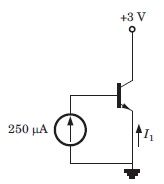Q. The base-emitter voltage is

Detailed Solution for Test: The Bipolar Junction Transistor - Question 16

The current source will forward bias the base-emitter junction and the collector base junction will then be reverse biased. Therefore the transistor is in the forward active region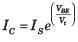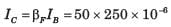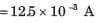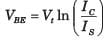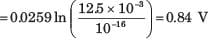Test: The Bipolar Junction Transistor - Question 17

For the circuit shown in fig., let the value of βR =0.5 and  βF = 50. The saturation current is 10-16 AQ. The current I1 is

Detailed Solution for Test: The Bipolar Junction Transistor - Question 17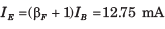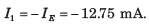Test: The Bipolar Junction Transistor - Question 18

The leakage current of a transistor are ICBO = 5μA and ICEO = 0.4 mA, and IB =30 μA

Q. The value of β is

Detailed Solution for Test: The Bipolar Junction Transistor - Question 18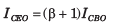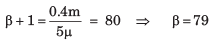Test: The Bipolar Junction Transistor - Question 19

The leakage current of a transistor are ICBO = 5μA and ICEO = 0.4 mA, and IB =30 μA

Q. The value of IC is

Detailed Solution for Test: The Bipolar Junction Transistor - Question 19

Ic = βIB + ICEO = 79(30μ) + 0.4m = 2.77 mA

Test: The Bipolar Junction Transistor - Question 20

For a BJT, IC = 5 mA, IB = 50 μA and ICBO = 0.5μA.

Q. The value of β is

Detailed Solution for Test: The Bipolar Junction Transistor - Question 20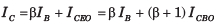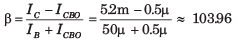## GATE Electrical Engineering (EE) 2023 Mock Test Series

18 docs|208 tests
 Use Code STAYHOME200 and get INR 200 additional OFF Use Coupon Code
Information about Test: The Bipolar Junction Transistor Page
In this test you can find the Exam questions for Test: The Bipolar Junction Transistor solved & explained in the simplest way possible. Besides giving Questions and answers for Test: The Bipolar Junction Transistor, EduRev gives you an ample number of Online tests for practice

## GATE Electrical Engineering (EE) 2023 Mock Test Series

18 docs|208 tests